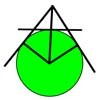#### You may also like### Mean Geometrically

A and B are two points on a circle centre O. Tangents at A and B cut at C. CO cuts the circle at D. What is the relationship between areas of ADBO, ABO and ACBO?### Without Calculus

Given that u>0 and v>0 find the smallest possible value of 1/u + 1/v given that u + v = 5 by different methods.### Pythagorean Golden Means

Show that the arithmetic mean, geometric mean and harmonic mean of a and b can be the lengths of the sides of a right-angles triangle if and only if a = bx^3, where x is the Golden Ratio.

# Three Ways

##### Age 16 to 18Challenge Level

Given that $x + y = -1$ find the largest value of $xy$
(a) by co-ordinate geometry
(b) by calculus
(c) by algebra.

Here are some solutions from Koopa,Boston College, USA. Vassil, Lawnswood Sixth Form, Leeds sent in similar methods. Can you find a co-ordinate geometry (i.e. graphical) method or yet another different method?

Method 1
We have $x + y = -1$. So, to maximise $xy$, I need to maximize

$-x(x + 1) = -[(x + 1/2)^2 - 1/4] = -(x + 1/2)^2 + 1/4,$

so, $xy$ is maximized at $x = -1/2$ and the maximum value is $1/4$.

Method 2
Let $f(x) = -x(x + 1)$, then by differentiation $f'(x) = -2x - 1$ and to find a maximum or minimum $f'(x)= 0$ gives $x = -1/2$. The second derivative test easily verifies that this indeed gives a maximum so the maximum value is $1/4$.

Method 3
By the AM-GM inequality, we have

$(xy)^{(1/2)} \leq (x + y)/2$

so $xy \leq (-1/2)^2 = 1/4.$